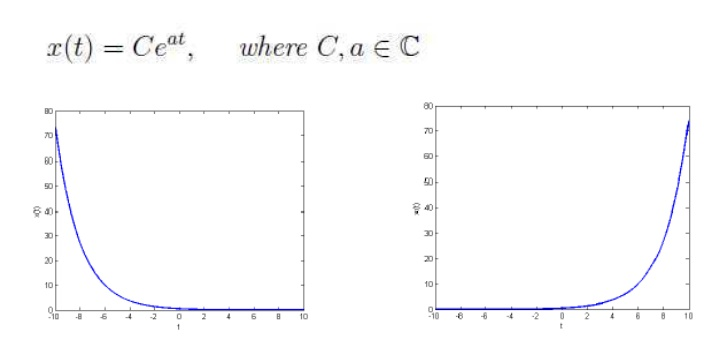Home | | Signals and Systems | Elementary Signals

# Elementary Signals

The elementary signals are used for analysis of systems. Such signals are, · Step · Impulse · Ramp · Exponential · Sinusoidal

Elementary Signals:

The elementary signals are used for analysis of systems. Such signals are,

·              Step

·              Impulse

·              Ramp

·              Exponential

·              Sinusoidal

1. Unit step signal:

·              Unit Step Sequence: The unit step signal has amplitude of 1 for positive value and amplitude of 0 for negative value of independent variable.

·              It have two different parameter such as CT unit step signal u(t) and DT unit step signal u(n).

·              The mathematical representation of CT unit step signal u(t) is given by,

2. Ramp Signal:

·              The amplitude of every sample is linearly increased with the positive value of independent variable.

·              Mathematical representation of CT unit ramp signal is given by,3. Unit impulse function:

Amplitude of unit impulse approaches 1 as the width approaches zero and it has zero value at all other values.

The mathematical representation of unit impulse signal for CT is given by,It is used to determine the impulse response of system.

4. Sinusoidal signal:

A continuous time sinusoidal signal is given by,5. Exponential signal:

·              It is exponentially growing or decaying signal.

·              Mathematical representation for CT exponential signal is,Study Material, Lecturing Notes, Assignment, Reference, Wiki description explanation, brief detail
Signals and Systems : Classification of Signals and Systems : Elementary Signals |